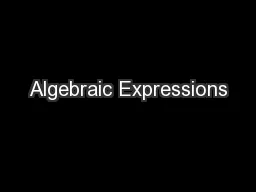/# Algebraic Expressions - PowerPoint Presentation

#### Algebraic Expressions - Description

Unit 7 Writing Algebraic Expressions with Addition and Subtraction Vocabulary Expressions A mathematical representation containing numbers variables and operation symbols an expression does not include an equality or inequality symbol ID: 540831 Download Presentation

#### Tags :

Download Presentation - The PPT/PDF document "Algebraic Expressions" is the property of its rightful owner. Permission is granted to download and print the materials on this web site for personal, non-commercial use only, and to display it on your personal computer provided you do not modify the materials and that you retain all copyright notices contained in the materials. By downloading content from our website, you accept the terms of this agreement.

#### Embed:

##### Presentation on theme: "Algebraic Expressions"— Presentation transcript

Slide1

Algebraic ExpressionsSlide2

Unit 7 – Writing Algebraic Expressions with Addition and SubtractionSlide3

Vocabulary

Expressions

- A mathematical representation containing numbers, variables, and operation symbols; an expression does not include an equality or inequality symbol.

Example

: (5+2)− 27 ÷3 (arithmetic/numerical expression)

2a +3b (algebraic/symbolic expression)

Terms

(of an expression)

– part of an algebraic expression separated by addition and/or subtraction

Example

: 9

w

2

+ 3

w

+10

in the expression above

there

are

3 terms, 9

w

2

, 3

w

and 10Slide4

Mathematical Phrases

A

d

d

i

t

ion

PlusAndTotal ofAltogetherIncreased byCombinedSumTogether**More than (flip-flopper)Added to (flip-flopper)In all

Subtraction

Subtract

Gave

Take away

Decreased by

Fewer

Minus

Fewer than (flip-flopper)

**Less than (flip-flopper)

Difference

Less

**Subtracted from (flip-flopper)Slide5

Writing Addition Algebraic Expressions - Example

Write an algebraic expression for the following scenario. Use a bar model to help you.

A box of unknown weight is added to a box whose weight is 10 kilograms. What is the total weight of the two boxes?

y

+ 10 kgSlide6

Writing Algebraic Addition Expressions - Example

Write an algebraic expression for the following scenario. Use a bar model to help you.

The length of a piece of wood is 9 centimeters. The length of another piece of wood is

x

centimeters. What is the total length of the two pieces of wood?

x

+ 9 cmSlide7

Write an algebraic expression for each of the following.

The sum of 7 and

j

.

7 +

jKent is 5 years older than Jake. What is Kent’s age in terms of y?

y + 5Michael is 3 years old and Jordan is p years old. What is their total age? 3 + pThe sum of

m

and 10.

m

+ 10Slide8

Writing Subtraction Algebraic Expressions - Example

Write an algebraic expression for the following scenario. Use a bar model to help you.

A shipping box containing a bowling ball has a total mass of 8 kilograms. If the shipping box weighs

m

kilograms, what is the weight of the bowling ball?

8

m

kgSlide9

Writing Subtraction Algebraic Expressions - Example

Write an algebraic expression for the following scenario. Use a bar model to help you.

Wally is 130 centimeters tall. Tia is

b

centimeters shorter than Wally. What is Tia’s height?

130 –

b

cmSlide10

Subtraction Algebraic Expression Practice

Write an algebraic expression for each of the following.

The difference of

a

and 53

a

-

53

Alexis had \$60 and she spent

t

dollars. How much money did she have left?

60

-

t

A small toy shop has

m

toys for sale in the store.

a. Tally buys 5 toys. Write an algebraic expression for the number of toys the shop has left.

m

– 5 toys

b. After Tally leaves the shop, Thomas buys 6 toys. Write an algebraic expression for how many toys the shop has left now.

m

- 11 toys